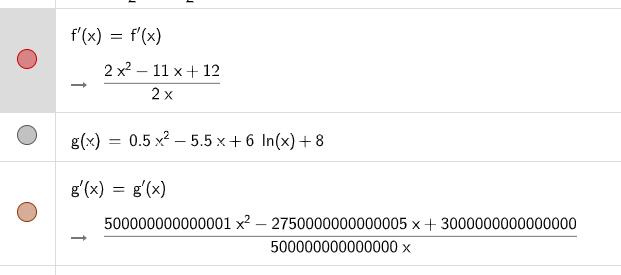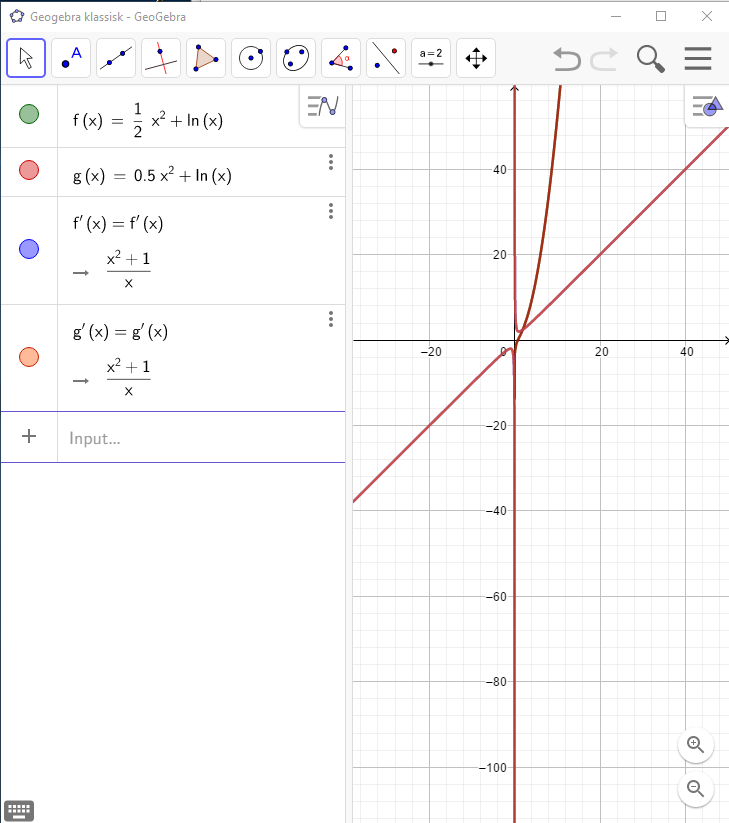# inconsistency with calculation of the derivative of a function with logarithm and decimal numbers

jjovuc shared this problem 2 years ago
Solved

Hi, I have encountered a problem when calculating the derivative of a function of decimal numbers and the natural logarithm. The problem occurs in geogebra classic 5 and the online classic app, but not in the downloadable app.

If I type a function given as 0.5x^2+ln(x) Geogebra accepts it as the same function as ½x^2+ln(x), but calculating the derivative functions returns two different functions as shown in this example (online version):

It seems that something weird happens when using decimal numbers. But this has already been fixed in the download version of Classic 6. Is it possible to fix the online app as well?1

Is this what you are seeing? GeoGebra works to 15 significant figures only if you enter decimals so I think it's OK1

We may have an improvement coming for this, I'll check.

Also you can try the CAS Calculator if you want exact answers eg https://www.geogebra.org/ca...1

Yes, that is what I'm seeing in Geogebra 5 and the online version. What confused me initially, is that Geogebra 6 download version returns the exact answer with the same setup, as shown below:1

Should be fixed now, please try!1

It seems to no longer be an issue, and the results are all consistent.

I tried the two examples above as well as other similar problems. Now both Geogebra 5 and the online version now gives the same exact results as the Geogebra 6 and online CAS version.

Thanks Michael!